# Money

Peter has 2x more money than Michael. But if Peter gave Michael € 16 Michael would have 3.5 times more money than Peter. Determine how much money was originally Peter and how much Michael.

Correct result:

m =  12
p =  24

#### Solution:

p=2m
3.5•(p-16) = (m+16)

p=2•m
3.5•(p-16) = (m+16)

2m-p = 0
m-3.5p = -72

m = 12
p = 24

Our linear equations calculator calculates it.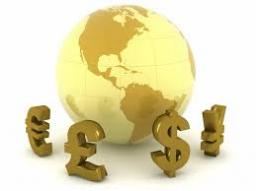We would be pleased if you find an error in the word problem, spelling mistakes, or inaccuracies and send it to us. Thank you!Tips to related online calculators
Do you have a linear equation or system of equations and looking for its solution? Or do you have quadratic equation?

## Next similar math problems:

• PotatoesDaniela and Michael would jointly dug potatoes for 7.5 hours. But if Daniela was working alone she would take 2.5 hours more as if he were working with Michael. Determine how much for the work done by Michael himself and how much Daniela herself.
• EurosPeter, Jane and Thomas have together € 550. Tomas has 20 euros more than Jane, Peter € 150 less than Thomas. Determine how much has each of them.
• RavensOn two trees sitting 17 ravens. If 3 ravens flew from first to second tree and 5 ravens took off from second tree then the first tree has 2 times more ravens than second tree. How many ravens was originally on every tree?
• Three brothersThe three brothers have a total of 42 years. Jan is five years younger than Peter and Peter is 2 years younger than Michael. How many years has each of them?
• Threesome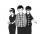Dana, Dalibor and Michael have a combined 57 years. Dana is five years older than Dalibor, but Dana is five years younger than Michael. Determine how old is Dana, Dalibor and Michael.
• Football seasonThroughout the football season gave Adam the 23 goals more than Brano and 16 goals less than Karol. Edo gave the 6 goals more Dušan. Karol gave 5 more than Edo. Brano and Edo gave together 17 goals. How many goals gave each boy and how many goals gave the
• NutsPeter has 49 nuts. Walnuts have 3 more than hazelnuts and hazelnut 2 over almonds. Determine the number of wallnuts, almonds and hazelnuts.
• Savings 2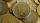Jozef and Michael saved 46 euros together. Michael saved 22 euros more than Jozef. How much did save each of them?
• Exam average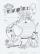Average of marks that have on the certificates students of 8.A class in mathematics is exactly 2.45. If we did not add 1 and 3 of sutdents Michael and Alena, who arrived a month ago, it would average exactly 2.5. Determine how many students have class 8.A
• Boys and money270 USD boys divided so that Peter got three times more than Paul and Ivan has 120 USD more than than Paul. How much each received?
• PearsAndrew, Lenka and Rasto have together 232 pears. Lenka has 28 more than Rasto and Rasto pears have 96 more than Andrew. Determine how much each of them has pears.
• 3 masons3 masons received 7,700 CZK. The second half received 1/2 more than the first and third twice more than the second mason. How much they each got crowns?
• More moneyLenka has €4.8 more than Natalia. Who will have more and how much, when Lenka give Natalia €2.5?
• The book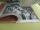If one day you read p pages, you will read the book 24 days. If you read each day 10 pages more, you would read a book in 16 days. How many pages have the book?
• Three workersThree workers were rewarded CZK 9200 and the money divided by the work they have done. First worker to get twice than the second, the second three times more than the third. How much money each worker received?
• Double ratio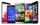The mobile phone was twice gradually discounted in the ratio of 3: 2 1 half: 5 quarters. How much did it originally cost if the price was CZK 4,200 after a double discount?
• Three friends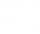Peter is eight times older than Rado, Rado is 3 times younger than Joseph. Together they have 120 years. Determine how many are each of them.